It’s easy to make a measurement in most instruments, but it’s sometimes hard to know what is real and what is an artifact. Gibbs Ringing is a common artifact in high-speed scope and TDR measurements that can lead you astray unless you understand how to avoid it. We reveal the secrets of avoiding this important artifact.

### Gibbs Ringing

An ideal square wave has a spectrum with harmonic amplitudes that drop off with 1/frequency. For an ideal square wave with a 0 psec rise time, the bandwidth is infinite, and we need every one of these harmonic components in the spectrum to recreate the 0 psec rise time.

When we synthesize a square wave in the time domain by adding successive sine waves as represented in its spectrum, we see a better and better approximation to the square wave as each harmonic is added. The rise time of the waveform gets shorter as we add harmonics and the waveform’s bandwidth increases. The top and bottom of the waveform begins to look flatter and flatter. However, there is a ringing artifact at the edges we cannot avoid. An example of a truncated series of sine waves including up to the 7th harmonic is shown in Figure 1.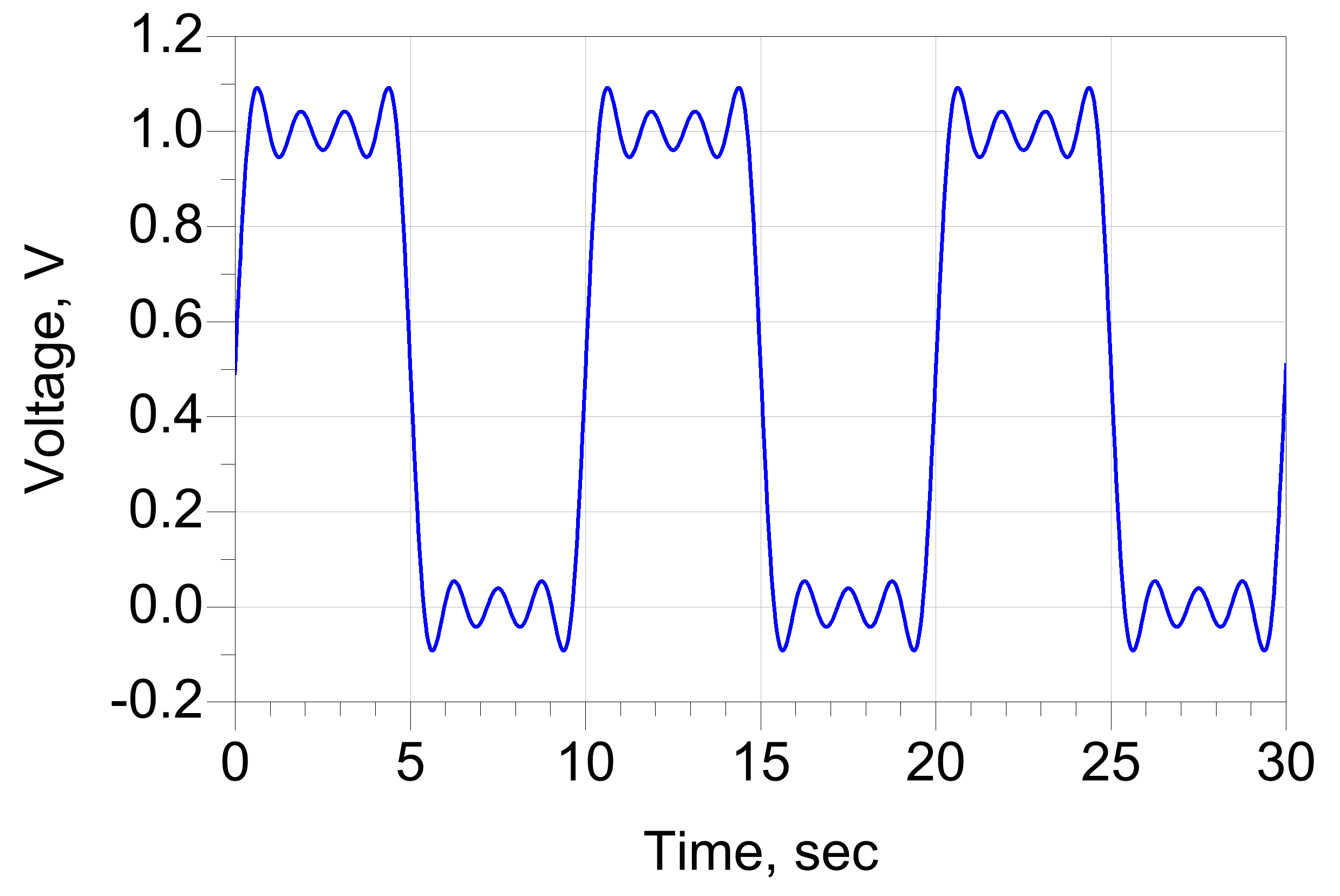Figure 1 The Waveform composed of just the first 7 harmonics of an ideal square wave.

This ringing behavior is observed in any waveform as the result of a sum of a truncated series of sine wave frequency components. In 1848, this behavior was first documented by Henry Wilbraham when synthesizing an ideal square wave from sine waves, which he did using pencil and paper calculation. In 1898, J Willard Gibbs reported on the ringing in a truncated Fourier expansion similar to Wilbraham’s observations. For historical reasons, this overshoot and ringing behavior became attributed to Gibbs and is a fundamental property of a truncated Fourier series. It is sometimes referred to as the Gibbs effect, the Gibbs phenomena, Gibbs ringing, or Gibbs ears.

Gibbs ringing will be observed whenever the infinite series of sine waves from an ideal square wave is truncated and transformed back to the time domain.

### Gibbs Ringing in the Step Response of Filters

Many instruments, such as high-speed scopes and even network analyzers, can be approximated as high order filters with a pole frequency and a filter order. Gibbs ringing will appear in the step response of a second order or higher filter. After all, in a step response, the input signal is a square wave composed of an infinite series of sine waves. The filter will truncate the infinite series of sine waves. Any sufficiently truncated series of sine waves will show Gibbs ringing.

In general, a filter is defined by its transfer function, H(f). This transfer function describes the complex ratio of the sine wave coming out to the sine wave going in. An example of the simulated transfer function of a 2-pole or 2nd order maximally flat low pass filter is shown in Figure 2.  All three important figures of merit are shown in the Figure 2.

```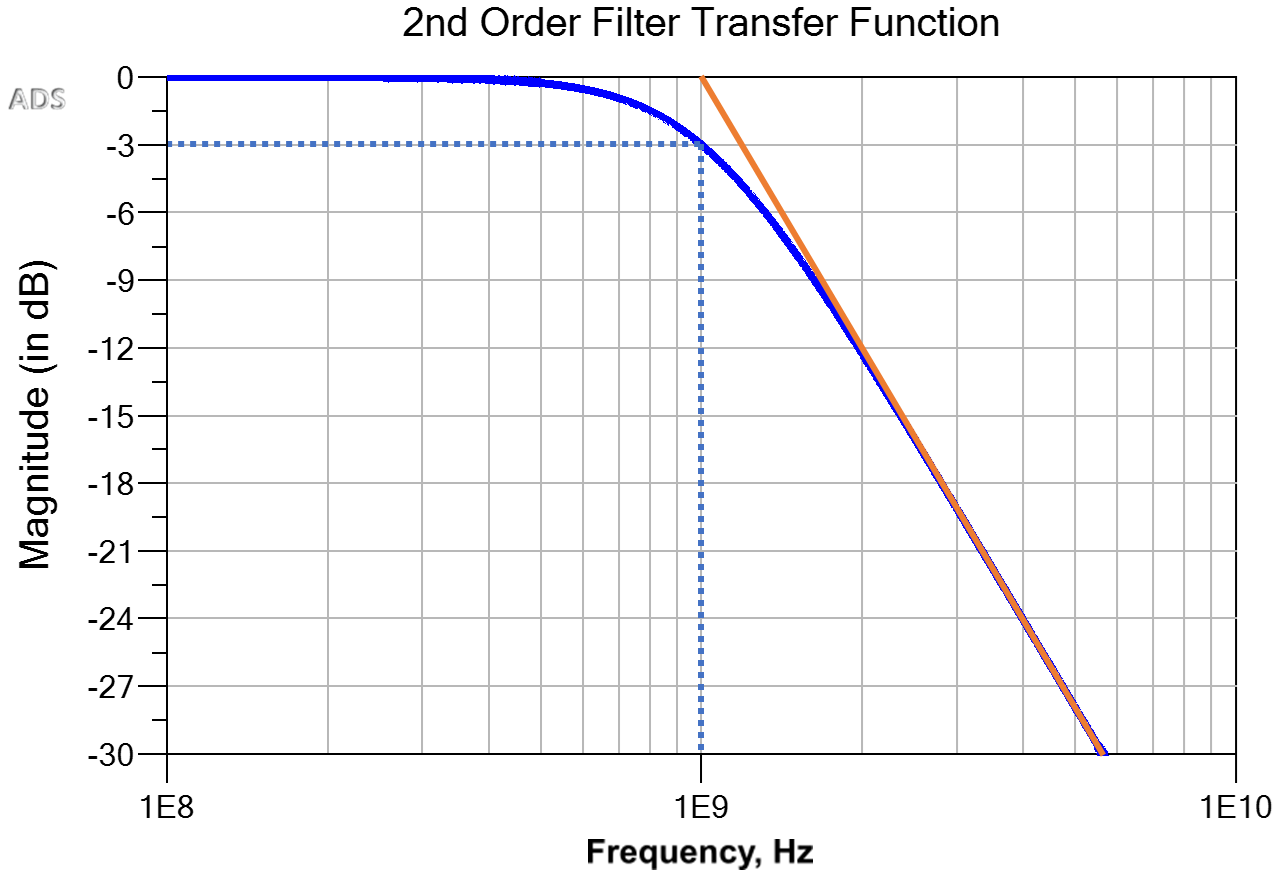```

Figure 2. Transfer function of a maximally flat 2nd order filter with a pole frequency of 1 GHz, and a passband level of 0 dB.The orange extrapolated slope of the decreasing magnitude hits the pass band magnitude at the pole frequency.

In a low pass filter, the transfer function is constant at low frequency, with a magnitude H0. This is the passband region.

The filter order is a measure of the shape of the transfer function and the number of reactive elements, such as L or C elements in its circuit description. It also describes how steeply the transfer function drops with frequency above the passband region. A 1st order filter will drop at a rate of -20 dB/decade of frequency increase, or -6 dB/octave of frequency increase. A 2nd order filter will drop at the rate of -40 dB/decade. An nth-order filter will drop at the rate of ([-20 x n] dB/decade). Higher order filters look more and more like a brick wall filter.

A filter’s step response is the output signal when the input signal is an ideal square wave. The step responses of different order filters, from 1st order to 8th order, are shown in Figure 3. In this example, the pole frequencies are all 1 GHz.

`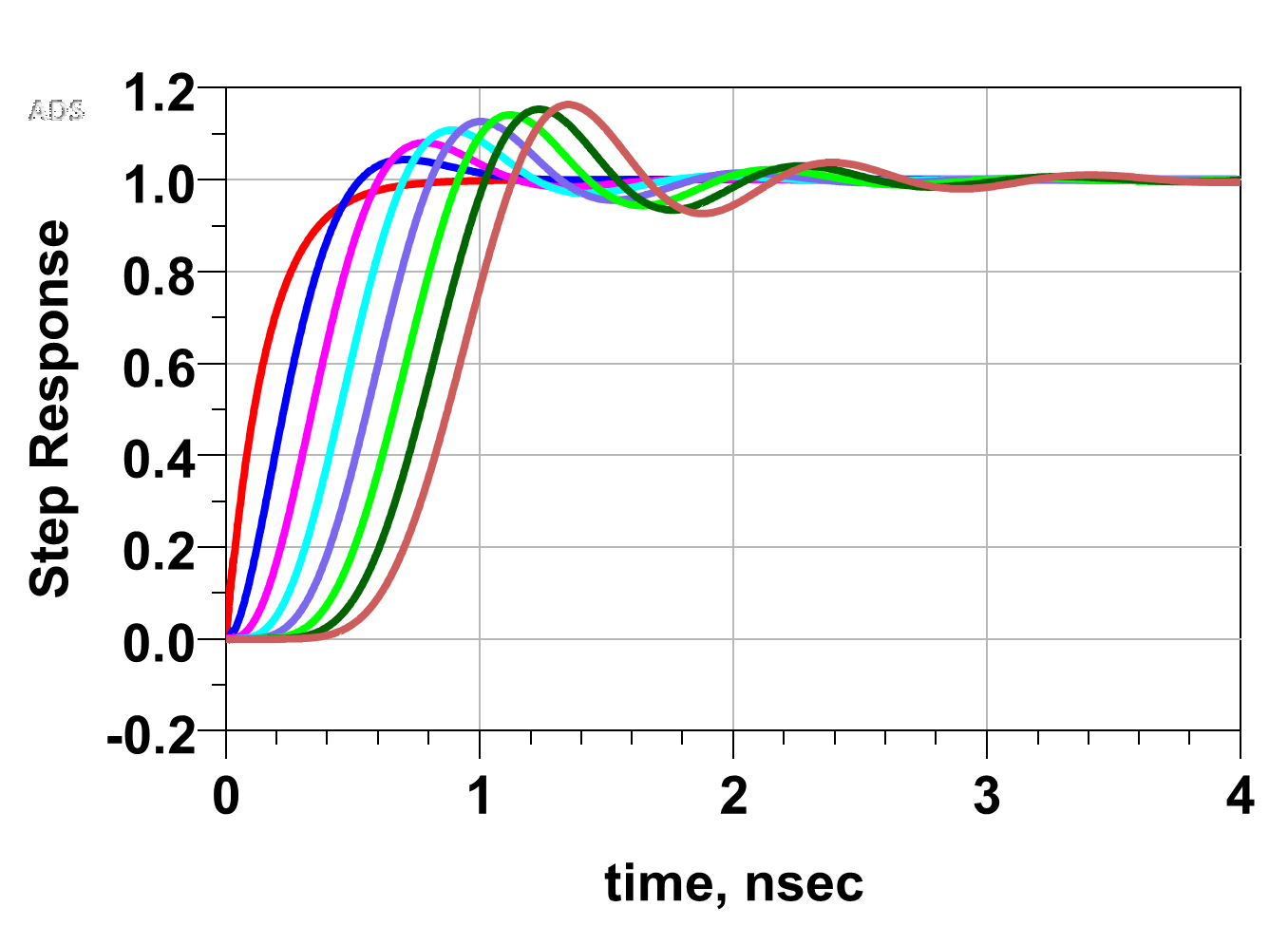`

Figure 3 The step responses of 1st to 8th order filters having the same pole frequency. The increasing delays with increasing filter order are due to the longer group delays of each filter.

A first order filter, the response of an RC circuit, does not show any Gibbs ringing. Its output exponentially decays to the 100% value. This suggests that a roll off of -20 dB/decade is not enough of a brick wall truncation to stimulate Gibbs ringing. However, the second order filter response and higher, does show Gibb’s ringing. The higher the order, the more pronounced the ringing amplitude. Afterall, the higher the order, the more of a brick wall the filter approximates.

The 10-90 rise times of each filter are the intrinsic rise time responses. Even though each filter has the same pole frequency of 1 GHz in this example, the intrinsic rise times are not the same. The 10-90 rise time and pole frequency are inversely related. An important figure of merit of any filter is the product of the intrinsic 10-90 rise time and the pole frequency, RTBW. This product depends only on the order of the filter. Figure 4a shows this relationship numerically simulated up to a 26th order filter.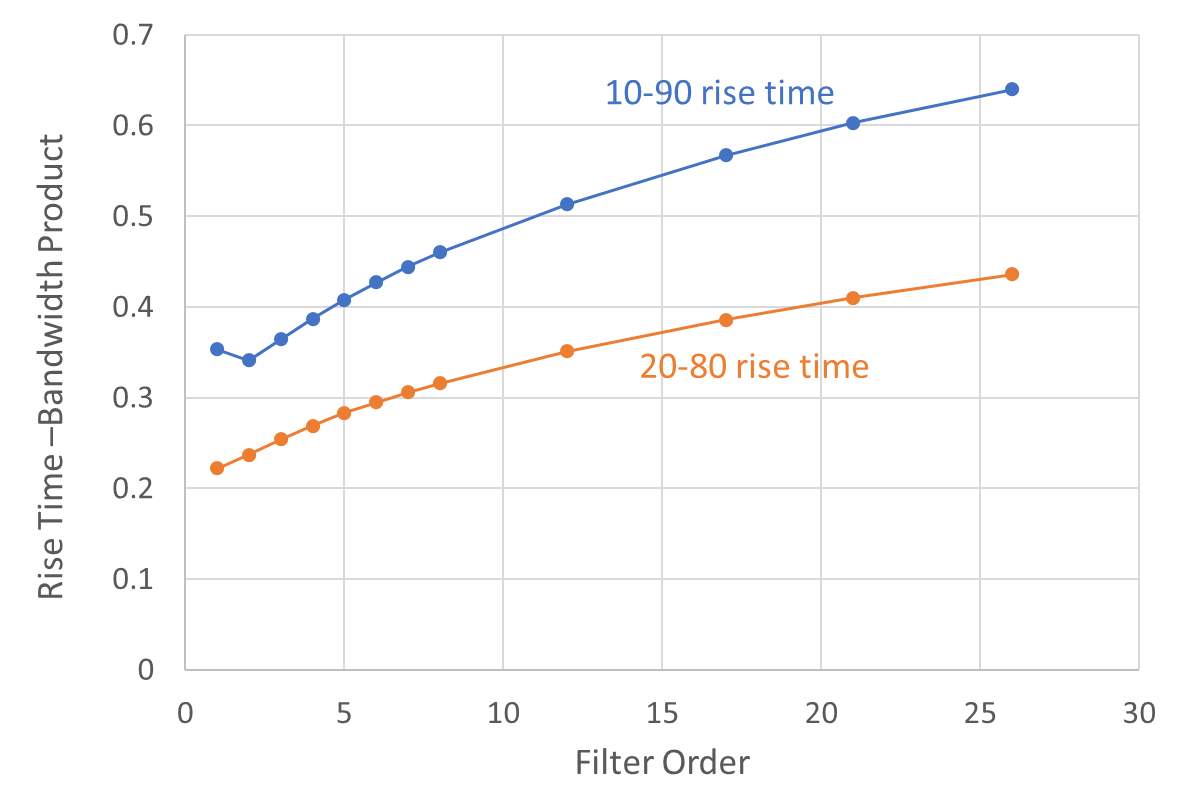Figure 4a Numerical experiment showing the extracted 10-90 rise time-bandwidth product for the step response of different ideal filters. The pole frequencies were the same for each filter. The 10-90 and 20-80 rise times show different Rise Time-Bandwidth products, but similar trends.

A 1st order filter has the well-known value of 0.35, while a 4th order filter has a RTBW product of 0.39 and an 8th order filter has a figure of merit of 0.46. If the bandwidth of a filter is known, this figure of merit can be used to estimate its intrinsic 10-90 rise time.

When an instrument behaves like a higher order filter, its step response will show a finite rise time, based on its pole frequency and filter order, and Gibbs ringing.

### Gibbs Ringing and Instrument Measurements

All modern high bandwidth scopes use extensive DSP filtering in their front ends. The filter order in the DSP algorithm is usually 4th order, but will vary depending on the scope settings, often times in ways that are hidden to the user. This means that their Rise Time-Bandwidth product is on the order of 0.39 to 0.45, depending on the setup, and they will show Gibbs ringing when excited by a signal with a bandwidth comparable to its pole frequency.

For example, a 50 psec rise time signal, from a low cost pulser purchased from Leo Bodnar Electronics for \$60, was used as a source. The bandwidth of this source is about 0.35/0.05 nsec = 7 GHz. It was measured with an 8 GHz and 4 GHz bandwidth scope. The intrinsic rise time of the scopes are about 0.39/8 GHz = 49 psec and 0.39/4 GHz = 98 psec respectively. These measurements were brought into Keysight’s ADS and compared with the response of an ideal 4th order maximally flat filter with a pole frequency of 8 GHz and 4 GHz using an ideal, 50 psec 10-90 rise time, error function, step edge stimulus. There was no fitting other than a time shift to align the edges. Figure 4b shows these measurements and the simulated ideal filter responses.

```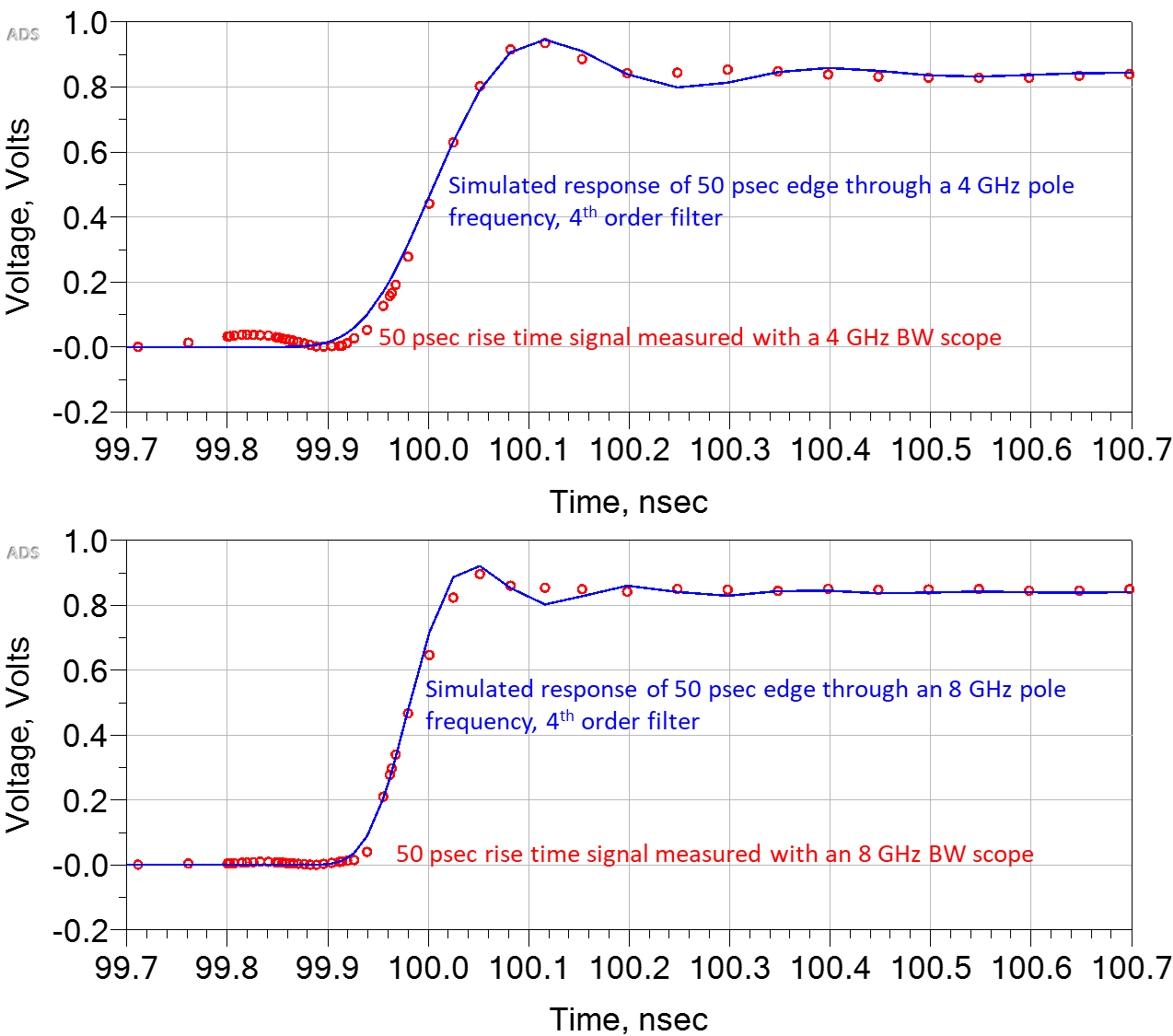```

Figure 4b Red circles: A 50 psec 10-90 rise time signal measured by two different Teledyne Lecroy scopes, with 4 GHz and 8 GHz bandwidth. The blue trace is the simulated response of a 50 psec signal through a 4th order filter with pole frequencies of 4 GHz and 8 GHz.

This overshoot and ringing can easily be misinterpreted as a real feature of the signal, such as a reflection feature, when in fact, it is an artifact of the response of the internal 4th order filter of the front end plus the DSP and the high bandwidth of the input signal. The DSP filter artificially truncated the input series of sine waves resulting in Gibbs ringing.

This is the same effect that occurs when transforming wide band S-parameter measurements into the time domain. The S-parameters are literally a truncated series of sine waves, truncated at the highest measured frequency in the S-parameter. When this band-limited series of sine waves is transformed into the time domain, Gibbs ringing is apparent.

The typical algorithm to translate band-limited S-parameters in the frequency domain to the time domain is to calculate the impulse response of the specific S-parameter by adding each sine wave component represented by the S-parameters. The truncated infinite series results in Gibbs ringing in the impulse response. The impulse response is then convolved with a synthesized input step signal to get the response of the interconnect in the time domain to a step edge.

A convolution of the impulse response and input waveform in the time domain is equivalent to the product of the frequency components of the input signal and the S-parameters in the frequency domain. When the input rise time is short enough that its bandwidth is comparable to the highest frequency in the S-parameters, the sine wave series of the signal will be truncated by the band-limited S-parameters and show Gibbs ringing in the time domain.

As an example, Figure 5 shows the time domain response of the transmitted signal through a very low loss interconnect, calculated from the frequency domain measurements, with measured frequency components up to 40 GHz. The step edge rise time was 9 psec, a bandwidth of 39 GHz. This left the S-parameters as a truncated series. Gibbs ringing is apparent.

```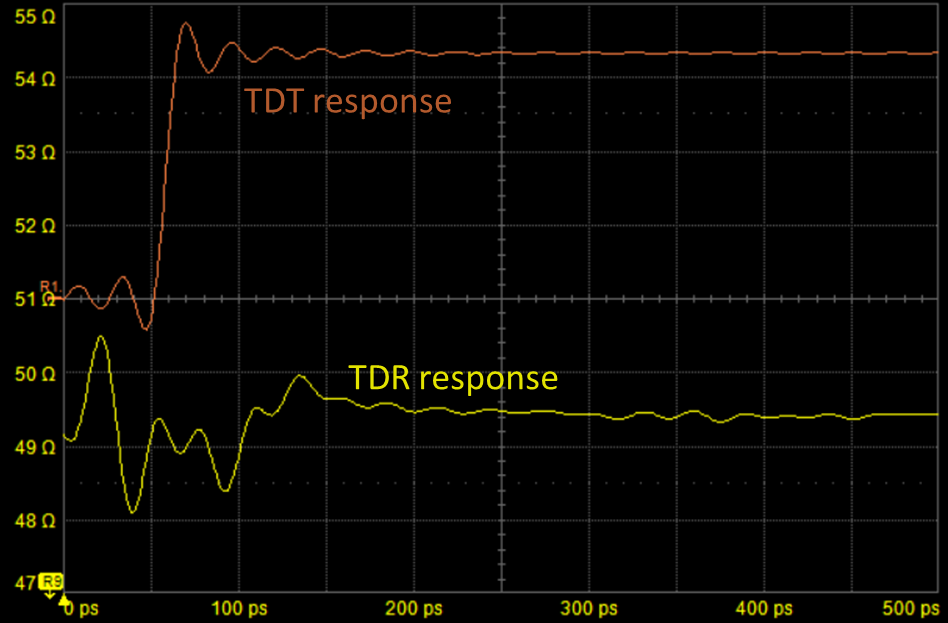```

Figure 5 Measured TDR and TDT of a short through interconnect with 40 GHz band limited S-parameters using too short a rise time in the recreated time domain response. The Gibbs effect artifact makes the interpretation of the response difficult.

When too short a rise time is used in the TDR measurement of an interconnect, created from frequency domain S-parameters, the Gibbs ringing artifact can make a uniform interconnect appear as an interconnect with discontinuities. Is this real or an artifact? How can this artifact be reduced or eliminated?

### Reducing Gibbs Ringing

The reason a 1st order filter does not show Gibbs ringing is that the -20 dB/decade drop in the amplitude of the sine waves is a gradual truncation. The higher the order of the filter, the higher the amplitude of Gibbs ringing because the truncation approximates more of a brick wall.

This suggests that if the infinite series of sine waves can be truncated gradually, within the range of frequencies used in the sum, rather than truncated as a brick wall, Gibbs ringing can be reduced. This means using bandwidth overhead to gradually reduce the truncation of the sine wave frequency components.

This principle is illustrated in Figure 6 where an increasing rise time step edge signal, with decreasing bandwidth, is used as an input to a 4th and 8th order filter. The input rise time, normalized to the intrinsic rise time of the filter, is used as a metric of the input rise time. When the input rise time is 2x the filter’s intrinsic rise time, there is little Gibbs ringing in the output of the filter, compared to when the input rise time is only 2% the intrinsic rise time.

```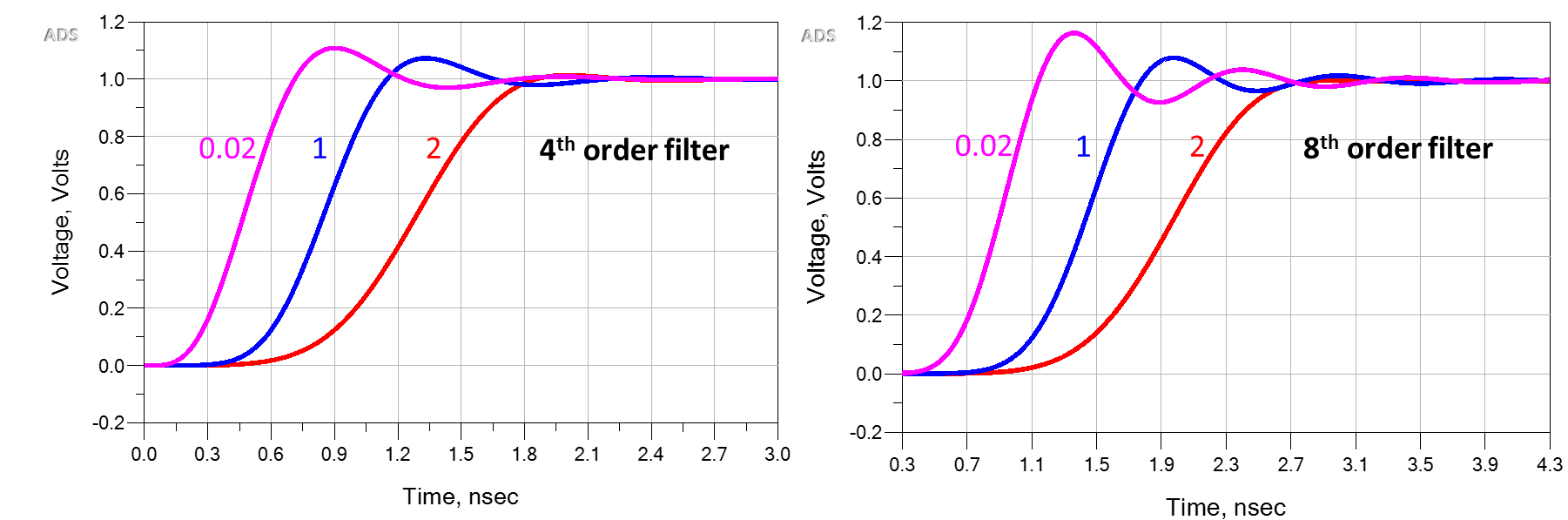```

Figure 6 Examples of Gibbs ringing at the output of a 4th order and 8th order filter at three different input risetimes, compared to the intrinsic system rise time of 0.02x, 1x and 2x. The overshoot value depends on the filter order and the input rise time compared to the filter’s intrinsic response.

The longer the rise time of the stimulus, compared to the intrinsic rise time of the filter, the less the impact from the Gibbs ringing in the output. Using a simple numerical experiment, we can answer the question of just how much longer the input rise time should be, compared to the intrinsic rise time, to eliminate the Gibbs ringing artifact.

Figure 7 shows the amount of Gibbs ringing overshoot in the filter’s response as the input rise time is increased, based on a numerical simulation. The normalized rise time to eliminate Gibbs ringing depends slightly on the order of the filter. For a 4th order filter, the rise time should be at least 2.5x the intrinsic rise time of the filter.